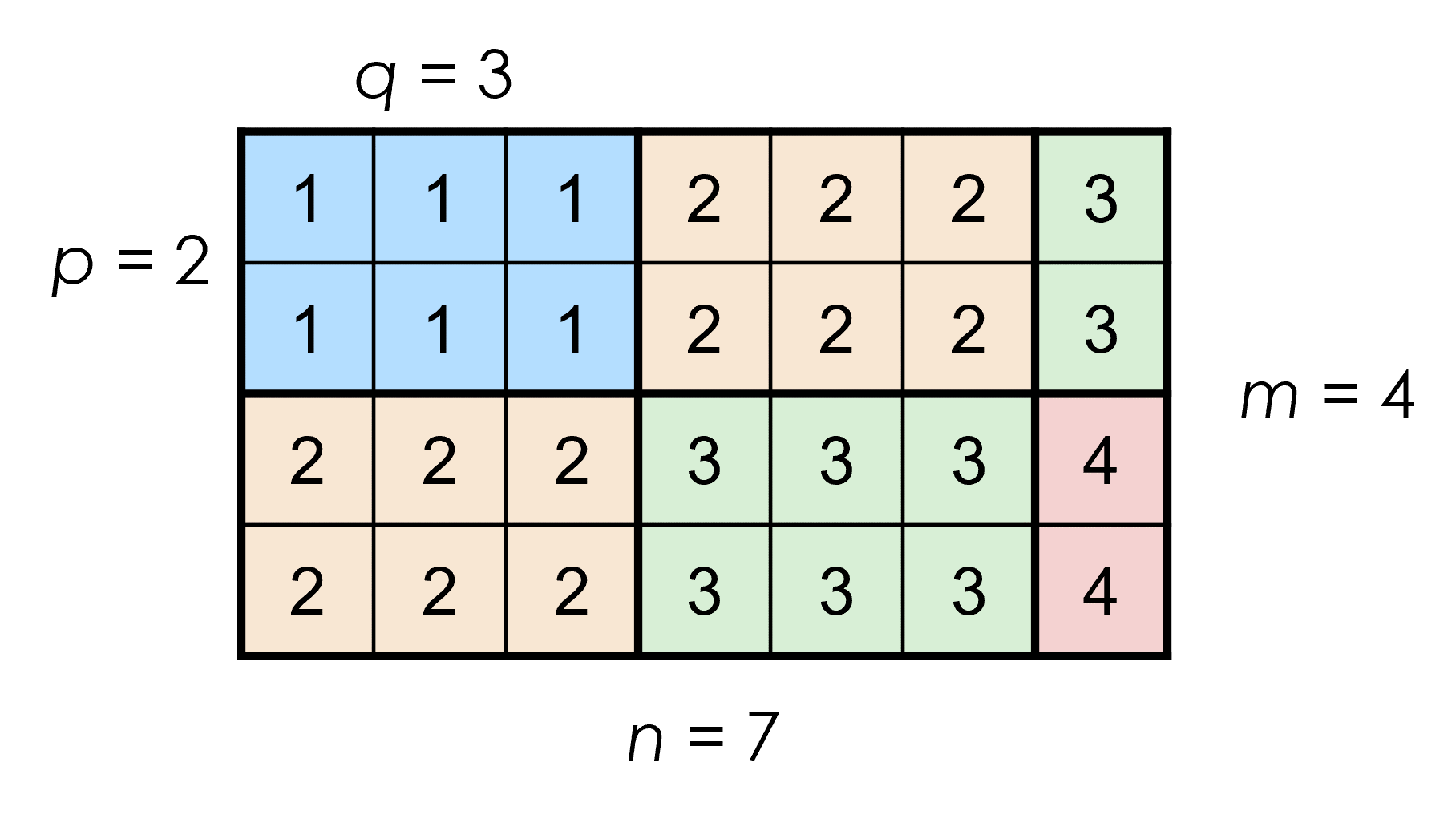# Problem 55260. Create block matrix of integers (j+k-1) - Part II

Given m, n, p, and q, create an m-by-n matrix made up of submatrices, each sized p-by-q (if possible - the last row and column of blocks may be smaller). The elements of the (j,k)th block all have the same value: (j+k-1).
For example, if m = 4, n = 7, p = 2, and q = 3, the matrix is:You can assume m, n, p, and q are all positive integers. (They can have the value 1, however.) As in the illustration above, m may or may not be divisible by p, and n may or may not be divisible by q. It is even possible for m < p or n < q. The resulting matrix will always be m-by-n.

### Solution Stats

57.69% Correct | 42.31% Incorrect
Last Solution submitted on Dec 07, 2023